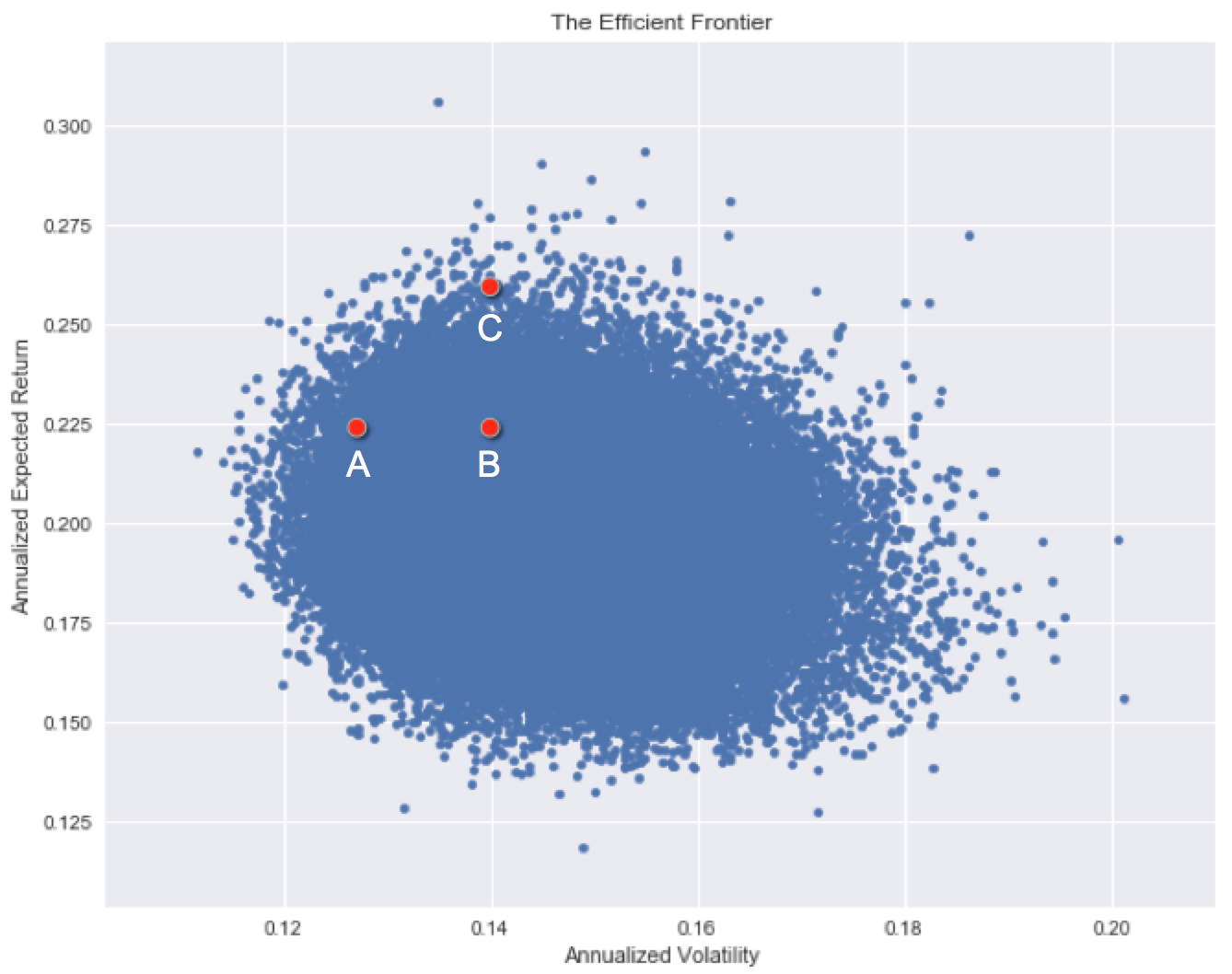# The efficient frontier

One of the ways to construct the efficient frontier of risk vs return given a set of assets is to randomly generate combinations and plot the characteristics of each portfolio. In this plot, the x-axis is the risk (standard deviation) and the y-axis is the expected return of each portfolio.Each investment has a historical risk level and a historical return. Note how some assets are more superior than others historically, but some are hard to choose between.

Which investment has the best historical risk / return characteristics?